# GMAT Math : DSQ: Understanding measurement

## Example Questions

2 Next →

### Example Question #11 : Dsq: Understanding Measurement

You are given a square metal sheet whose area can be given by a whole number of square yards. What is the length of each side?

Statement 1: The area of the sheet is less than 3,000 square inches.

Statement 2: The area of the sheet is less than 15 square feet.

BOTH statements TOGETHER are sufficient to answer the question, but NEITHER statement ALONE is sufficient to answer the question.

BOTH statements TOGETHER are insufficient to answer the question.

Statement 1 ALONE is sufficient to answer the question, but Statement 2 ALONE is NOT sufficient to answer the question.

EITHER statement ALONE is sufficient to answer the question.

Statement 2 ALONE is sufficient to answer the question, but Statement 1 ALONE is NOT sufficient to answer the question.

Statement 2 ALONE is sufficient to answer the question, but Statement 1 ALONE is NOT sufficient to answer the question.

Explanation:

Assume Statement 1 alone. One yard is equal to 36 inches, so one square yard is equal to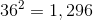square inches. The maximum area of 3,000 square inches is equal to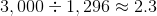square yards; since the area is a whole number of square yards less than this, we can narrow the area down to 1 or 2 square yards. Without further information, we cannot narrow this down further.

Assume Statement 2 alone. One yard is equal to 3 feet, so one square yard is equal to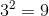square feet. The maximum area of 15 square feet is equivalent to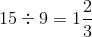square yards; since the area is a whole number of square yards less than this, the only possible area is one square yard.

### Example Question #11 : Measurement Problems

The length of a metal rod can be given by a whole number of inches. How long is the rod?

Statement 1: The length of the rod can be given by a whole number of feet.

Statement 2: The rod is between 40 and 50 inches long, inclusive.

Statement 1 ALONE is sufficient to answer the question, but Statement 2 ALONE is NOT sufficient to answer the question.

Statement 2 ALONE is sufficient to answer the question, but Statement 1 ALONE is NOT sufficient to answer the question.

BOTH statements TOGETHER are sufficient to answer the question, but NEITHER statement ALONE is sufficient to answer the question.

BOTH statements TOGETHER are insufficient to answer the question.

EITHER statement ALONE is sufficient to answer the question.

BOTH statements TOGETHER are sufficient to answer the question, but NEITHER statement ALONE is sufficient to answer the question.

Explanation:

If Statement 1 alone is assumed, since the length of the rod is given by a whole number of feet, then the length in inches must be a multiple of 12 - 12 inches, 24 inches, etc. However, no other information can be found.

If Statement 2 alone is assumed, there are eleven possible lengths of the rod - 40 inches, 41 inches, and so on up to 50 inches.

Now assume both statements. The length of the rod in inches must be a multiple of 12 between 40 and 50; this narrows it down to one possibility, 48 inches.

### Example Question #111 : Word Problems

You are given a square metal sheet whose area can be given by a whole number of square meters. What is the length of each side?

Statement 1: The length of each side is between 250 and 280 centimeters, inclusive.

Statement 2: The length of each side is between 2,500 millimeters and 2,800 millimeters inclusive.

BOTH statements TOGETHER are sufficient to answer the question, but NEITHER statement ALONE is sufficient to answer the question.

Statement 2 ALONE is sufficient to answer the question, but Statement 1 ALONE is NOT sufficient to answer the question.

BOTH statements TOGETHER are insufficient to answer the question.

EITHER statement ALONE is sufficient to answer the question.

Statement 1 ALONE is sufficient to answer the question, but Statement 2 ALONE is NOT sufficient to answer the question.

EITHER statement ALONE is sufficient to answer the question.

Explanation:

Assume Statement 1 alone. Since one meter is equal to 100 centimeters, the minimum and maximum lengths in meters are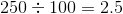meters and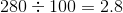meters. The squares of these lengths provide the minimum and maximum areas of the sheet: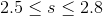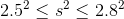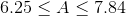From the main body of the problem, the area in square meters must be a whole number, so its area must be seven meters.

Assume Statement 2 alone. One meter is equal to 1,000 millimeters, so the minumum and maximum lengths are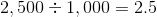meters and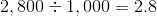meters. These are the same boundaries derived from Statement 1, so again, the area can be determined to be seven meters.

2 Next →

### All GMAT Math Resources